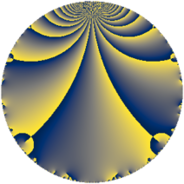# Properties

 Label 175.3.dLevel $175$ Weight $3$ Character orbit 175.d Rep. character $\chi_{175}(76,\cdot)$ Character field $\Q$ Dimension $23$ Newform subspaces $10$ Sturm bound $60$ Trace bound $2$

# Related objects

## Defining parameters

 Level: $$N$$ $$=$$ $$175 = 5^{2} \cdot 7$$ Weight: $$k$$ $$=$$ $$3$$ Character orbit: $$[\chi]$$ $$=$$ 175.d (of order $$2$$ and degree $$1$$) Character conductor: $$\operatorname{cond}(\chi)$$ $$=$$ $$7$$ Character field: $$\Q$$ Newform subspaces: $$10$$ Sturm bound: $$60$$ Trace bound: $$2$$ Distinguishing $$T_p$$: $$2$$, $$3$$

## Dimensions

The following table gives the dimensions of various subspaces of $$M_{3}(175, [\chi])$$.

Total New Old
Modular forms 46 29 17
Cusp forms 34 23 11
Eisenstein series 12 6 6

## Trace form

 $$23 q + q^{2} + 49 q^{4} - 3 q^{7} + 5 q^{8} - 69 q^{9} + O(q^{10})$$ $$23 q + q^{2} + 49 q^{4} - 3 q^{7} + 5 q^{8} - 69 q^{9} - 18 q^{11} - 15 q^{14} + 113 q^{16} - 11 q^{18} + 12 q^{21} - 10 q^{22} - 86 q^{23} + 77 q^{28} + 102 q^{29} + 21 q^{32} - 375 q^{36} + 66 q^{37} + 204 q^{39} - 60 q^{42} + 174 q^{43} - 192 q^{44} + 144 q^{46} - 121 q^{49} + 324 q^{51} - 74 q^{53} - 321 q^{56} - 180 q^{57} - 370 q^{58} + 233 q^{63} - 185 q^{64} + 86 q^{67} - 438 q^{71} + 245 q^{72} - 504 q^{74} - 74 q^{77} + 300 q^{78} + 142 q^{79} + 87 q^{81} + 792 q^{84} + 864 q^{86} - 62 q^{88} - 468 q^{91} + 66 q^{92} - 660 q^{93} + 409 q^{98} + 426 q^{99} + O(q^{100})$$

## Decomposition of $$S_{3}^{\mathrm{new}}(175, [\chi])$$ into newform subspaces

Label Dim $A$ Field CM Traces $q$-expansion
$a_{2}$ $a_{3}$ $a_{5}$ $a_{7}$
175.3.d.a $1$ $4.768$ $$\Q$$ $$\Q(\sqrt{-7})$$ $$3$$ $$0$$ $$0$$ $$7$$ $$q+3q^{2}+5q^{4}+7q^{7}+3q^{8}+9q^{9}+\cdots$$
175.3.d.b $2$ $4.768$ $$\Q(\sqrt{-10})$$ None $$-6$$ $$0$$ $$0$$ $$6$$ $$q-3q^{2}+\beta q^{3}+5q^{4}-3\beta q^{6}+(3+2\beta )q^{7}+\cdots$$
175.3.d.c $2$ $4.768$ $$\Q(\sqrt{-5})$$ None $$-4$$ $$0$$ $$0$$ $$4$$ $$q-2q^{2}+\beta q^{3}-2\beta q^{6}+(2-3\beta )q^{7}+\cdots$$
175.3.d.d $2$ $4.768$ $$\Q(\sqrt{21})$$ $$\Q(\sqrt{-7})$$ $$-3$$ $$0$$ $$0$$ $$14$$ $$q+(-1-\beta )q^{2}+(2+3\beta )q^{4}+7q^{7}+\cdots$$
175.3.d.e $2$ $4.768$ $$\Q(\sqrt{-1})$$ $$\Q(\sqrt{-35})$$ $$0$$ $$0$$ $$0$$ $$0$$ $$q+iq^{3}-4q^{4}-7iq^{7}+8q^{9}-13q^{11}+\cdots$$
175.3.d.f $2$ $4.768$ $$\Q(\sqrt{-5})$$ None $$2$$ $$0$$ $$0$$ $$-14$$ $$q+q^{2}+\beta q^{3}-3q^{4}+\beta q^{6}-7q^{7}+\cdots$$
175.3.d.g $2$ $4.768$ $$\Q(\sqrt{21})$$ $$\Q(\sqrt{-7})$$ $$3$$ $$0$$ $$0$$ $$-14$$ $$q+(1+\beta )q^{2}+(2+3\beta )q^{4}-7q^{7}+(13+\cdots)q^{8}+\cdots$$
175.3.d.h $2$ $4.768$ $$\Q(\sqrt{-10})$$ None $$6$$ $$0$$ $$0$$ $$-6$$ $$q+3q^{2}+\beta q^{3}+5q^{4}+3\beta q^{6}+(-3+\cdots)q^{7}+\cdots$$
175.3.d.i $4$ $4.768$ 4.0.1163520.6 None $$-4$$ $$0$$ $$0$$ $$4$$ $$q+(-1+\beta _{3})q^{2}-\beta _{1}q^{3}+(3-2\beta _{3})q^{4}+\cdots$$
175.3.d.j $4$ $4.768$ 4.0.1163520.6 None $$4$$ $$0$$ $$0$$ $$-4$$ $$q+(1-\beta _{3})q^{2}-\beta _{1}q^{3}+(3-2\beta _{3})q^{4}+\cdots$$

## Decomposition of $$S_{3}^{\mathrm{old}}(175, [\chi])$$ into lower level spaces

$$S_{3}^{\mathrm{old}}(175, [\chi]) \cong$$ $$S_{3}^{\mathrm{new}}(7, [\chi])$$$$^{\oplus 3}$$$$\oplus$$$$S_{3}^{\mathrm{new}}(35, [\chi])$$$$^{\oplus 2}$$